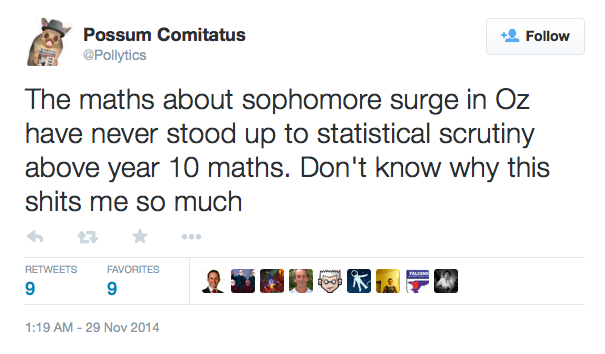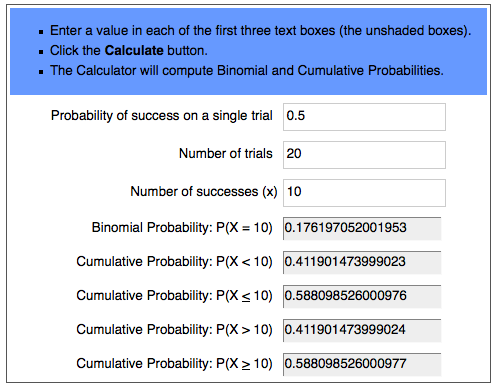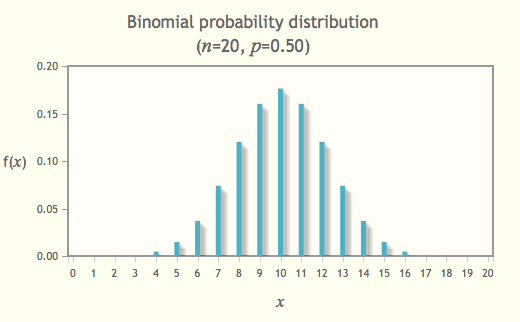Tuesday, 24 March 2015

The Sophomore Surge

I'm a regular reader of Peter Brent (@mumbletwits on Twitter) and his blog posts in The Australian newspaper. He talks regularly about politics and, in particular, psephology, which is the study of elections and trends in voting. One aspect of Mumble's writing I particularly like is that he remains, in his writing, non-partisan, something more opinion writers and journalists could learn to do.  Maybe it's just that all politicians give him the shits.

Sophomore Surge

One particular idea that Brent discusses regularly in analysing election results is the Sophomore Surge.  In summary, the Sophomore Surge encapsulates the idea that a member newly elected at one election gains an advantage at the next election due to the fact that, in the meantime, they gather a personal following of people who vote for them not due to their party, but due to the relationship they build up with individual voters with whom they interact and work.  This tends to be a one-off effect for any parliamentarian.  Let's face it, if you haven't warmed to your local member after the first three years, you're unlikely to do so later.  There's a bit more to it than that, but you can read more about it from Brent directly here.

Not all psephologists agree that this concept has any significant bearing on election outcomes, with one particularly ardent critic being Possum Commitatus (@pollytics on Twitter).  His opinion generally goes along the lines belowFigure 1: Tweet by @pollytics
Now, while I can't answer his last question (I suspect it may actually be rhetorical), I do have the capacity to use statistics above a year 10 level to analyse election results, and determine whether, statistically speaking, the Sophomore Surge has a significant effect on election outcomes for those candidate.  As a maths teacher, I hope I can also explain it well for the layperson & laypossums (laypossa?).

Don't be scared by the maths

The maths is actually at a Year 12 level, and I'll present the whole argument below logically so anyone, regardless of ability with a pencil, should be able to follow along.  The actual tricky calculations that this task requires can all be done using online calculators, so mostly the sort of maths you need to follow this is logic.  If you're still reading at this point, you'll be fine with the maths.  If you're starting to think tl;dr, maybe this site would be more interesting.

Setting up the problem - the data

The data I have chosen to use in the analysis below is from the last Federal Election, held in September 2013.  Why?  It's fairly recent, and the data is easy to get.  I did start looking at the Victorian State election, but there have been a significant number of redistributions since the previous election, making it difficult to really identify any true Sophomore candidates.  There were very few changes in electoral boundaries between the 2007 and 2013 federal elections that had an impact on our Sophomore candidates.  There are are also more seats federally, so a larger data set.  (As it turns out, this data set is still not large enough, but will do for the purpose of explaining how this process works - other, more serious, psephologists may wish to attack this problem with bigger data sets if they wish).

Setting up the problem - definitions

We also need to define which candidates we're interested in, so we're all on the same page.
We're interested only in politicians facing their second consecutive election.  So those elected for the first time in 2010, and faced re-election in 2013 for the first time.  It doesn't matter whether they won in 2013 or not.  These are the Sophomore candidates for 2013.
What we want to test is whether their new incumbency gives them a better result than the general election results across the whole country.

The way I'm going to measure this is to compare the swing each Sophomore candidate had at the 2013 election to the overall national swing.  Now, in 2013, the national swing was 3.65% towards the Coalition, so for a Sophomore candidate from a Coalition Party (Libs, Nats, LNP, CLP), a swing of greater than 3.65% would count as doing better, while a swing of 3.65% or less would result in not doing better.  For an ALP Sophomore candidate, it would be the opposite (ie swing of less than 3.65% towards the Coalition is good, 3.65% or more towards the Coalition is bad).

Binomial Statistics - simple as flipping a coin

Measuring the outcome in terms of only two possible results (better than average or not better than average) is an important part of the problem.  It allows us to treat this as a binomial problem (bi meaning two). A bit like flipping a coin - the result is either heads or tails.

If you flip a coin 20 times, the most likely result will be 10 heads and 10 tails, but lets be honest, we wouldn't be surprised if it was 11 to 9, or even 12 to 8.  What about 13 to 7? 15 to 5?  At what point do we start getting suspicious that the coin is rigged?

Fortunately, binomial statistics allows us to calculate exactly how likely a specific outcome is, and there are plenty of online calculators that do the hard work for us  We just have to know how to put the data in.  All you need is three values.
• The number of trials, n (how many coin tosses)
• The probability of success as a fraction of 1, p (in the case of a fair coin, 1/2 or 0.5)
• The number of successes, k (how meany heads)
Using the calculator linked above, you can see that the chance of getting exactly ten heads in twenty tosses (written as P(X=10)) is about 17.6% (multiply the Binomial Probability decimal value of 0.1716... from the table below by 100 to turn it into a percentage).  We'll look at the Cumulative Probabilities and what they mean a little later

Here's how it looks on the websiteFigure 2: Probability of flipping 20 coins and getting 10 heads

If you graph the probability of each possible outcome from zero heads to twenty heads out of twenty tosses, and you get the well known bell curve below (figure 3, calculated from a different online calculator).  You can see the most likely outcome is 10 heads, although 9 or 11 would not be unusual.Figure 3: Binomial distribution for flipping a coin 20 times
Using the same online calculator, we can calculate that the probability of getting 12 heads in 20 coin flips.
By looking at the Binomial Probability P(X=12), we can see that probability of getting 12 heads in 20 flips is approximately 12%.

Testing a Hypothesis

The normal way mathematicians do this is to set up a hypothesis that can be tested.  In this case, we could define the hypothesis H0 as The Coin is Fair, and the alternative hypothesis H1 as The Coin is Not Fair.  We then look at the data to determine the probability of getting our result or something even more unusual, assuming that H0 is in fact true.  If it is less than some arbitrary value, often 5%, then we say that is unlikely that our hypothesis, H0, is true, and therefore H1 must be true instead.

Testing our coin hypothesis, the probability of getting 12 heads or more, is identified in the above table by the symbols P(X>=12), which has a value of approximately 25%.  What this means is that we could expect to get 12 or more heads in 20 coin flips, approximately 25% of the time.  This is reasonably likely, or at least higher than our 5% standard, and so we have to assume the H0 is true, that is, that the coin is fair.

(If you play around with the online calculator you can easily see that in order to get the Cumulative Probability down below 5%, you would need to get 15 heads or more in 20 flips)

Counter intuitively, these results  don't scale.  What this means is that while 12 heads out of 20 is consistent with a fair coin, 120 heads out of 200 isn't.  In fact the probability of getting 120 or more heads out of 200 flips is less than 0.3%, well below our standard of 5%.

The Actual Data

In 2013 there were 26 candidates who won in 2010 as new candidates and ran for relection.  Of these 26, I am going to exclude six as not being simple cases.  These six are
• Warren Entsch in Leichardt, Qld
• Ross Vast in Bonner, Qld
• Teresa Gambaro in Brisbane, Qld
• Rob Mitchell in McEwan, Vic
• Louise Markus in Macquarie, NSW
• Laurie Ferguson in Werriwa, NSW
The reason for excluding the first three is that they were all members previous to 2007, so that by and large the conditions for a Sophomore Surge won't necessarily apply, ie, the electorate already knows them.  Rob Mitchell had never been the local member, but had been the candidate several times, making it difficult to determine if the Sophomore effect would be important or not.  The last two were affected by redistributions between 2010 and 2013, so I excluded them as well.  They may get half a Sophomore Surge. Best to leave them out.

This leaves us with 20 ridgy-didge Sophomore candidates to play around with. (I've lost the original table, but can redo if anyone is particularly interested)
Of the 20 candidates, 12 had a swing that was better than the national average. If there is no Sophomore Surge, then we would expect that there was a 50% chance that any particular candidate would receive an above average swing, and 50% chance they wouldn't, and the most likely outcome would be 10 with a better than average result, and 10 less.  But is 12 with an above average swing really that unusual or unexpected?

Funnily enough(!), the maths here works out to be exactly the same as in our example of a coin above.  Of the 20 Sophomore candidates (n=20), 12 gained swings better than the national average (k=12), where it is expected that there is a 50% chance (p=0.5) of an above swing.  As in the above example, let's set our Hypothesis, H0, to be There is no Sophomore Effect, with the alternate hypothesis, H1, The Sophomore Effect Holds.

Assuming that H0 is true, there is no Sophomore effect, then there is a 25% probability that 12  or more of our Sophomore candidates would receive better than average swings.  As this is a reasonably high probability (higher than 5%), our data indicates that H0, There is no Sophomore Surge, is an acceptable explanation for the result given.

More data needed!

As we saw above, though, more data can easily give a better picture (and a sample of 20 is really quite small).  The technique used here can easily be applied to data aggregated across a large number of elections.  The process to do so is remarkably easy.
• For n, add the total number of Sophomore Candidates across a set of elections
• For k, add the total number of Sophomore Candidates who achieved a better than average swing in the relevant election
• For p, always use 0.5%

Hasn't anyone already thought of doing this?

Well, yes.  A quick google search on the term Sophomore Surge will provide links to a number of  research papers like this one, using much more sophisticated maths than I have, that tend to support the existence and significance of the Sophomore Surge

PS this blog was mostly written before the Qld state election, and was intended to be published before then.  I got busy :)  With the NSW state election coming up this weekend, it seemed appropriate to publish it now.  Perhaps after this weekend, I'll gather data form these two state elections and beef up the stats.

1.2.